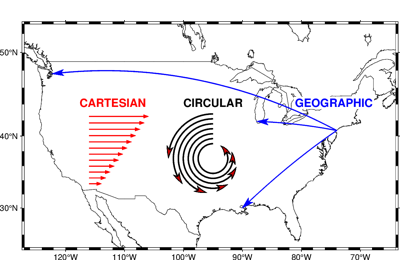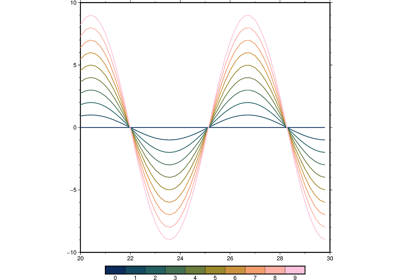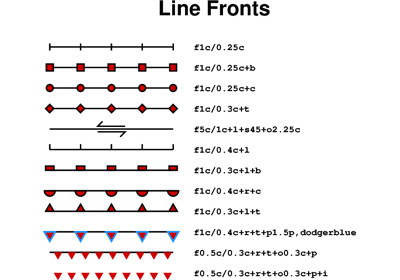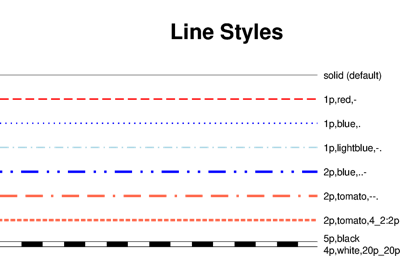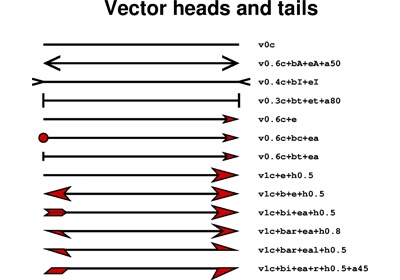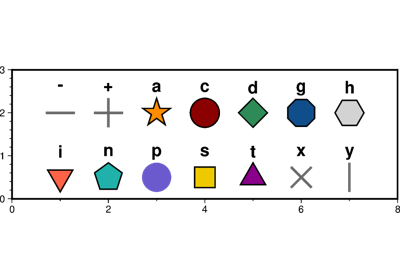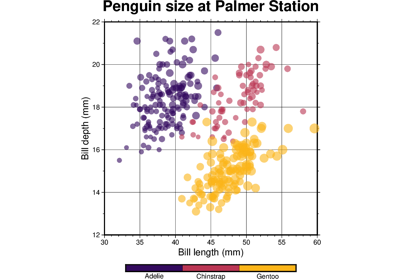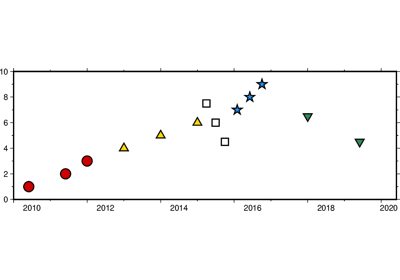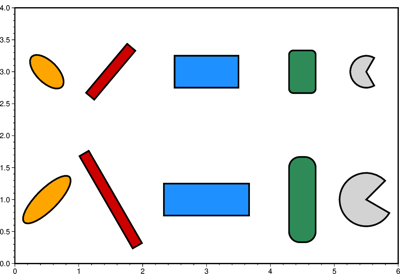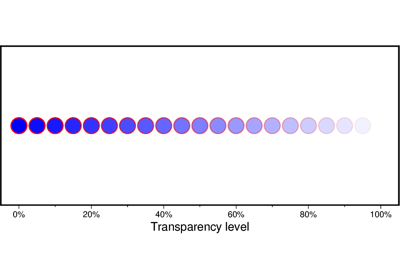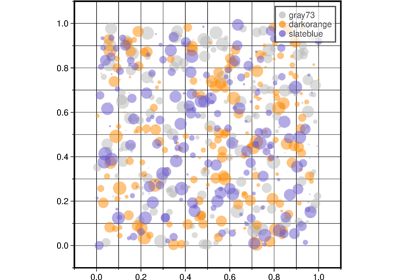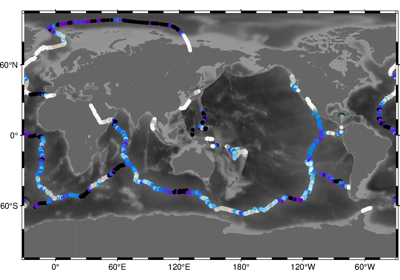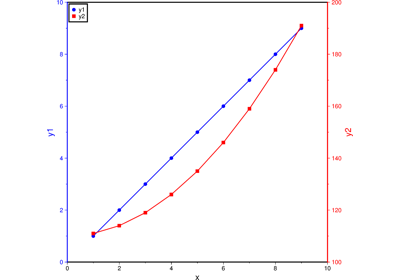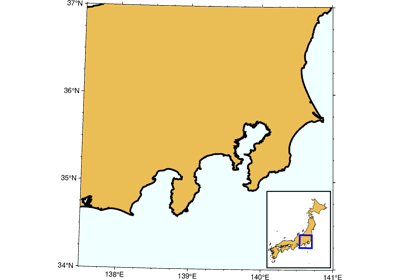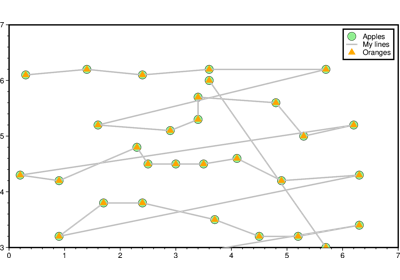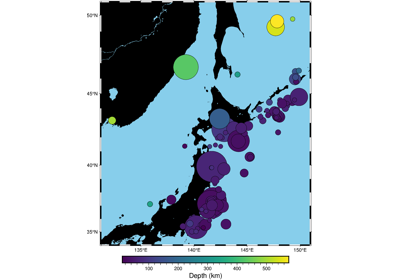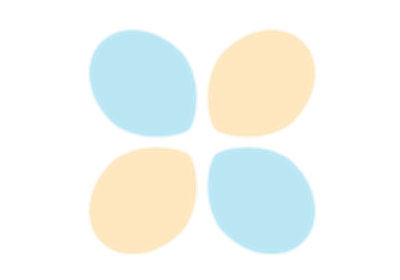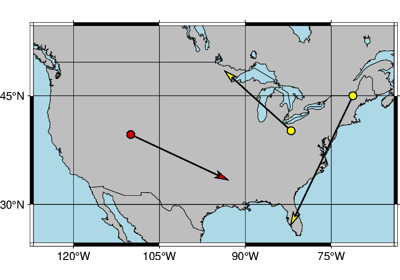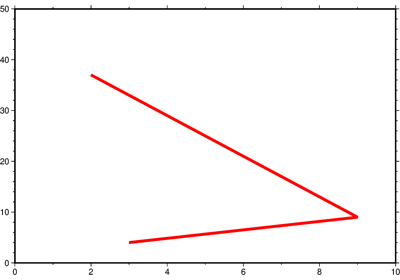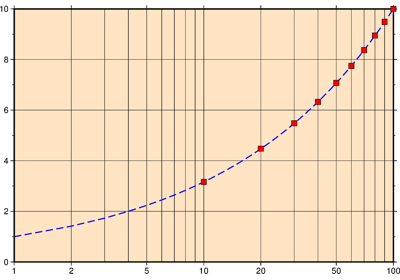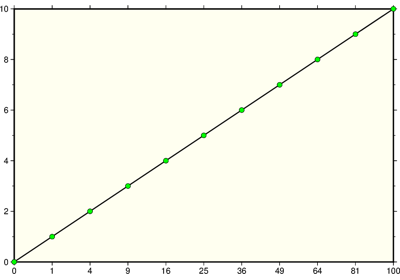# pygmt.Figure.plot¶

Figure.plot(x=None, y=None, data=None, size=None, direction=None, *, straight_line=None, frame=None, cmap=None, offset=None, error_bar=None, connection=None, color=None, intensity=None, projection=None, close=None, no_clip=None, region=None, style=None, timestamp=None, verbose=None, pen=None, xshift=None, yshift=None, zvalue=None, aspatial=None, incols=None, label=None, panel=None, coltypes=None, perspective=None, transparency=None, **kwargs)

Plot lines, polygons, and symbols in 2-D.

Takes a matrix, (x,y) pairs, or a file name as input and plots lines, polygons, or symbols at those locations on a map.

Must provide either `data` or `x`/`y`.

If providing data through `x`/`y`, `color` can be a 1d array that will be mapped to a colormap.

If a symbol is selected and no symbol size given, then plot will interpret the third column of the input data as symbol size. Symbols whose size is <= 0 are skipped. If no symbols are specified then the symbol code (see `style` below) must be present as last column in the input. If `style` is not used, a line connecting the data points will be drawn instead. To explicitly close polygons, use `close`. Select a fill with `color`. If `color` is set, `pen` will control whether the polygon outline is drawn or not. If a symbol is selected, `color` and `pen` determines the fill and outline/no outline, respectively.

Full parameter list at https://docs.generic-mapping-tools.org/latest/plot.html

Aliases:

• A = straight_line

• B = frame

• C = cmap

• D = offset

• E = error_bar

• F = connection

• G = color

• I = intensity

• J = projection

• L = close

• N = no_clip

• R = region

• S = style

• U = timestamp

• V = verbose

• W = pen

• X = xshift

• Y = yshift

• Z = zvalue

• a = aspatial

• c = panel

• f = coltypes

• i = incols

• l = label

• p = perspective

• t = transparency

Parameters
• x/y (float or 1d arrays) – The x and y coordinates, or arrays of x and y coordinates of the data points

• data (str or numpy.ndarray or pandas.DataFrame or xarray.Dataset or geopandas.GeoDataFrame) – Pass in either a file name to an ASCII data table, a 2D `numpy.ndarray`, a `pandas.DataFrame`, an `xarray.Dataset` made up of 1D `xarray.DataArray` data variables, or a `geopandas.GeoDataFrame` containing the tabular data. Use parameter `incols` to choose which columns are x, y, color, and size, respectively.

• size (1d array) – The size of the data points in units specified using `style`. Only valid if using `x`/`y`.

• direction (list of two 1d arrays) – If plotting vectors (using `style='V'` or `style='v'`), then should be a list of two 1d arrays with the vector directions. These can be angle and length, azimuth and length, or x and y components, depending on the style options chosen.

• projection (str) – Required if this is the first plot command. projcode[projparams/]width. Select map projection.

• region (str or list) – Required if this is the first plot command. xmin/xmax/ymin/ymax[+r][+uunit]. Specify the region of interest.

• straight_line (bool or str) – [m|p|x|y]. By default, geographic line segments are drawn as great circle arcs. To draw them as straight lines, use `straight_line`. Alternatively, add m to draw the line by first following a meridian, then a parallel. Or append p to start following a parallel, then a meridian. (This can be practical to draw a line along parallels, for example). For Cartesian data, points are simply connected, unless you append x or y to draw stair-case curves that whose first move is along x or y, respectively.

• frame (bool or str or list) – Set map boundary frame and axes attributes.

• cmap (str) – File name of a CPT file or a series of comma-separated colors (e.g., color1,color2,color3) to build a linear continuous CPT from those colors automatically.

• offset (str) – dx/dy. Offset the plot symbol or line locations by the given amounts dx/dy [Default is no offset]. If dy is not given it is set equal to dx.

• error_bar (bool or str) – [+b|d|D][+xl|r|x0][+yl|r|y0][+ppen]. Draw symmetrical error bars. Full documentation is at https://docs.generic-mapping-tools.org/latest/plot.html#e.

• connection (str) –

[c|n|r][a|f|s|r|refpoint]. Alter the way points are connected (by specifying a scheme) and data are grouped (by specifying a method). Append one of three line connection schemes:

• c : Draw continuous line segments for each group [Default].

• r : Draw line segments from a reference point reset for each group.

• n : Draw networks of line segments between all points in each group.

Optionally, append the one of four segmentation methods to define the group:

• a : Ignore all segment headers, i.e., let all points belong to a single group, and set group reference point to the very first point of the first file.

• f : Consider all data in each file to be a single separate group and reset the group reference point to the first point of each group.

• s : Segment headers are honored so each segment is a group; the group reference point is reset to the first point of each incoming segment [Default].

• r : Same as s, but the group reference point is reset after each record to the previous point (this method is only available with the `connection='r'` scheme).

Instead of the codes a|f|s|r you may append the coordinates of a refpoint which will serve as a fixed external reference point for all groups.

• color (str or 1d array) – Select color or pattern for filling of symbols or polygons. Default is no fill. color can be a 1d array, but it is only valid if using `x`/`y` and `cmap=True` is also required.

• intensity (float or bool or 1d array) – Provide an intensity value (nominally in the -1 to +1 range) to modulate the fill color by simulating illumination. If using `intensity=True`, we will instead read intensity from the first data column after the symbol parameters (if given). intensity can also be a 1d array to set varying intensity for symbols, but it is only valid for `x`/`y` pairs.

• close (str) – [+b|d|D][+xl|r|x0][+yl|r|y0][+ppen]. Force closed polygons. Full documentation is at https://docs.generic-mapping-tools.org/latest/plot.html#l.

• no_clip (bool or str) – [c|r]. Do NOT clip symbols that fall outside map border [Default plots points whose coordinates are strictly inside the map border only]. The parameter does not apply to lines and polygons which are always clipped to the map region. For periodic (360-longitude) maps we must plot all symbols twice in case they are clipped by the repeating boundary. `no_clip=True` will turn off clipping and not plot repeating symbols. Use `no_clip="r"` to turn off clipping but retain the plotting of such repeating symbols, or use `no_clip="c"` to retain clipping but turn off plotting of repeating symbols.

• style (str) – Plot symbols (including vectors, pie slices, fronts, decorated or quoted lines).

• pen (str) – Set pen attributes for lines or the outline of symbols.

• timestamp (bool or str) – Draw GMT time stamp logo on plot.

• verbose (bool or str) –

Select verbosity level [Default is w], which modulates the messages written to stderr. Choose among 7 levels of verbosity:

• q - Quiet, not even fatal error messages are produced

• e - Error messages only

• w - Warnings [Default]

• t - Timings (report runtimes for time-intensive algorithms);

• i - Informational messages (same as `verbose=True`)

• c - Compatibility warnings

• d - Debugging messages

• xshift (str) – [a|c|f|r][xshift]. Shift plot origin in x-direction.

• yshift (str) – [a|c|f|r][yshift]. Shift plot origin in y-direction. Full documentation is at https://docs.generic-mapping-tools.org/latest/gmt.html#xy-full.

• zvalue (str) – value|file. Instead of specifying a symbol or polygon fill and outline color via `color` and `pen`, give both a value via `zvalue` and a color lookup table via `cmap`. Alternatively, give the name of a file with one z-value (read from the last column) for each polygon in the input data. To apply it to the fill color, use `color='+z'`. To apply it to the pen color, append +z to `pen`.

• aspatial (bool or str) – [col=]name[,…]. Control how aspatial data are handled during input and output. Full documentation is at https://docs.generic-mapping-tools.org/latest/gmt.html#aspatial-full.

• panel (bool or int or list) – [row,col|index]. Select a specific subplot panel. Only allowed when in subplot mode. Use `panel=True` to advance to the next panel in the selected order. Instead of row,col you may also give a scalar value index which depends on the order you set via `autolabel` when the subplot was defined. Note: row, col, and index all start at 0.

• coltypes (str) – [i|o]colinfo. Specify data types of input and/or output columns (time or geographical data). Full documentation is at https://docs.generic-mapping-tools.org/latest/gmt.html#f-full.

• incols (str or 1d array) –

Specify data columns for primary input in arbitrary order. Columns can be repeated and columns not listed will be skipped [Default reads all columns in order, starting with the first (i.e., column 0)].

• For 1d array: specify individual columns in input order (e.g., `incols=[1,0]` for the 2nd column followed by the 1st column).

• For `str`: specify individual columns or column ranges in the format start[:inc]:stop, where inc defaults to 1 if not specified, with columns and/or column ranges separated by commas (e.g., `incols="0:2,4+l"` to input the first three columns followed by the log-transformed 5th column). To read from a given column until the end of the record, leave off stop when specifying the column range. To read trailing text, add the column t. Append the word number to t to ingest only a single word from the trailing text. Instead of specifying columns, use `incols="n"` to simply read numerical input and skip trailing text. Optionally, append one of the following modifiers to any column or column range to transform the input columns:

• +l to take the log10 of the input values.

• +d to divide the input values by the factor divisor [Default is 1].

• +s to multiple the input values by the factor scale [Default is 1].

• +o to add the given offset to the input values [Default is 0].

• label (str) – Add a legend entry for the symbol or line being plotted. Full documentation is at https://docs.generic-mapping-tools.org/latest/gmt.html#l-full.

• perspective (list or str) – [x|y|z]azim[/elev[/zlevel]][+wlon0/lat0[/z0]][+vx0/y0]. Select perspective view and set the azimuth and elevation angle of the viewpoint. Default is [180, 90]. Full documentation is at https://docs.generic-mapping-tools.org/latest/gmt.html#perspective-full.

• transparency (int or float) – Set transparency level, in [0-100] percent range. Default is 0, i.e., opaque. Only visible when PDF or raster format output is selected. Only the PNG format selection adds a transparency layer in the image (for further processing). transparency can also be a 1d array to set varying transparency for symbols, but this option is only valid if using x/y.

## Examples using `pygmt.Figure.plot`¶